# Gibbs Sampling Algorithm Implementation for Searching DNA Motifs

Sequence motifs are knobs and switches that allow organisms to control levels of RNA and protein products. They work as handles for regulatory proteins to interact with the sequences they control. They are also a primary target for evolutionary processes to act on to fine-tune the phenotypes.

## 1. Intro

You can test it out yourself at this notebook.

This is a simple implementation of Gibbs motif sampler algorithm described in class 7.91 lecture 9. Gibbs motif sampler is a kind of Monte Carlo algorithm which relies on repeated random sampling of data. Algorithm is implemented in pure python and pandas and matplotlib only used to plot the results. Below code sets up the virtual environment.

```virtualenv venv
. venv/bin/activate
pip install -U matplotlib pandas
```

The newest function in use probably is random.choices which is implemented in python 3.6 so this code should work any version after python 3.6 (at least up to 3.10).

```import sys
import pandas as pd
import matplotlib as pyplot

print("python", sys.version)
print("pandas", pd.__version__)
print("matplotlib", pyplot.__version__)
```
```python 3.10.8 (main, Nov  4 2022, 09:21:25) [GCC 12.2.0]
pandas 1.5.1
matplotlib 3.6.2
```

## 2. Implementation

### 2.1. Creating the sample data

#### 2.1.1. Creating Sequences

We can create our test data by using random module. First lets create some sequences. For this we need 4 variables:

• Alphabet which is A, G, C, T for DNA
• Weights for our bases meaning how frequent we are gonna see them
• How long we want our sequences to be
```alphabet = ['A', 'C', 'G', 'T']
seq_weights = [.25, .25, .25, .25]
seq_length = 60
```

We can loop over the number of sequences we want and use the random.choices to pick bases which returns a list that we can join into a string. Lets create 10 random sequences.

```import random

for _ in range(10):
seq = "".join(
random.choices(population=alphabet, weights=seq_weights, k=seq_length)
)
print(seq)
```
```TTCCGCTCCGTGCTGCTTTTCGTGCACGTTCTCTGAGCTGACTACTAGATTCACGTAGGG
TGTGGCGCGCAAGGCATTTTTTGCGCTTTGTGCGTTTAGCGTAGAATCTAAGAGTGGAGG
GCCTAATAAATTACACAGCAGAATACGTTAGTAGTCCGCACCGGCCTCGAGCACATCCCT
GGGCCGAACAGCTGCCGCGACCAGCGTTCCCTTTATGAGTCGCAGATGAAGTCTATCACC
TTAGGCTCAAGGTTTAGGGGTGCGAGAACTGCGAATCCGCCAAAGACCCATCTTCCGCCG
TTGCTGAAGAACGCCGGCCTCTCCATGTCGACAATAAACGATTACGTCTCCCGAGACTTT
AACTTGGTAATAAAATCAAGTGTGGGTTTGTAGGTCTCGTTGAAGCATTCCTAGACTAAC
CTGGATCGCACAAGGAGCCTCTGCGCAGGTATTTTGTGTATCTCTAATCTTGGAATTTGC
CACGTTCATGACGATTGAACACATTATAAGTAACAGTAACTATCCCTTGATAGATTTCAT
CCAAAAACGGACGACCTACCGCACCTTCGGTCCTCACTACGCAAGGACTGGAGCGTATCT
```

#### 2.1.2. Creating motifs

There is a standard definition from UIPAC to represent multiple bases in single character. In order the create a motif I want to enter a motif logo that is represented by UIPAC codes like `ABCDMRS` with weights for possible bases in each location.

We can represent the UIPAC codes in our program as a simple dictionary.

```IUPACcodes = {
"A": ["A"],
"C": ["C"],
"G": ["G"],
"T": ["T"],
"M": ["A", "C"],
"R": ["A", "G"],
"W": ["A", "T"],
"S": ["C", "G"],
"Y": ["C", "T"],
"K": ["G", "T"],
"V": ["A", "C", "G"],
"H": ["A", "C", "T"],
"D": ["A", "G",
"B": ["C", "G", "T"],
"N": ["A", "C", "G", "T"],
}
```

To create a motif we will need two parameters:

• motif logo
• and respective weight of the bases

In example below, first list of `` corresponds to first `A` in the logo. Second list of `[0.8, 0.2]` corresponds to code `K` which stands for either G or T. Here 0.8 is weight of the base G and 0.2 is weight of the base T.

```motif_logo = 'AKCYTSG'
motif_weights = [
,
[0.8, 0.2],
,
[0.8, 0.2],
,
[0.8, 0.2],
,
]
```

We can now generate random motifs with some variability. Here we loop over the logo translate them to the bases and pick one of the bases with given weights using random.choices.

```def generate_motif(logo, weights):
"""
Create a random motif from motif logo.
"""
motif = ""
for index, code in enumerate(logo):
bases = IUPACcodes[code]
motif = "".join(
(
motif[:index],
random.choices(bases, weights[index]),
motif[index + 1 :],
)
)
return motif

```

Lets create 10 logos.

```for _ in range(10):
motif = generate_motif(motif_logo, motif_weights)
print(motif)
```
```AGCCTGG
AGCCTCG
AGCCTCG
AGCCTCG
AGCCTCG
AGCCTCG
AGCCTCG
AGCCTCG
AGCCTCG
AGCCTGG
```

#### 2.1.3. Adding motifs to sequences

Lastly we insert the motifs randomly into the sequences. Here we return the `insert_ind` so we can see where we inserted the motifs.

```def insert_motif(sequence, motif):
'''
Replace a random part of the given sequence with the given motif sequence
'''
insert_ind = random.randrange(len(sequence) - len(motif))
sequence = ''.join((sequence[:insert_ind], motif, sequence[insert_ind + len(motif):]))
return insert_ind, sequence
```

#### 2.1.4. Putting it all together

Lets create 120 sequences and print first 10 of them. Here we can align using the `insert_ind` returned by `insert_motif` function to see the motifs clearly. Also to make the sample data reproducible we set random.seed here so we get the same sequences with same motifs inserted in the same positions.

```import random
random.seed(0)

"""
Takes IUPAC code returns a list of possible bases.
"""
IUPACcodes = {
"A": ["A"],
"C": ["C"],
"G": ["G"],
"T": ["T"],
"M": ["A", "C"],
"R": ["A", "G"],
"W": ["A", "T"],
"S": ["C", "G"],
"Y": ["C", "T"],
"K": ["G", "T"],
"V": ["A", "C", "G"],
"H": ["A", "C", "T"],
"D": ["A", "G", "T"],
"B": ["C", "G", "T"],
"N": ["A", "C", "G", "T"],
}

def generate_motif(logo, weights):
"""
Create a random motif from motif logo.
"""
motif = ""
for index, code in enumerate(logo):
bases = IUPACcodes[code]
motif = "".join(
(
motif[:index],
random.choices(bases, weights[index]),
motif[index + 1 :],
)
)
return motif

def insert_motif(sequence, motif):
'''
Replace a random part of the given sequence with the given motif sequence
'''
insert_ind = random.randrange(len(sequence) - len(motif))
sequence = ''.join((sequence[:insert_ind], motif, sequence[insert_ind + len(motif):]))
return insert_ind, sequence

alphabet = ['A', 'C', 'G', 'T']
seq_weights = [.25, .25, .25, .25]
seq_length = 60
num_seqs = 120

motif_logo = 'AKCYTSG'
motif_weights = [
,
[0.8, 0.2],
,
[0.8, 0.2],
,
[0.8, 0.2],
,
]

motif_seqs = []
for i in range(num_seqs):
seq = "".join(
random.choices(population=alphabet, weights=seq_weights, k=seq_length)
)
motif_seq = generate_motif(motif_logo, motif_weights)
motif_seqs.append(insert_motif(seq, motif_seq))

print(len(motif_seqs) * " " + motif_logo)
for insert_ind, sequence in motif_seqs[:10]:
print((len(sequence) - insert_ind) * " " +sequence)
```
```                                                            AKCYTSG
TTCCGCTCCGTGCTGCTTTTCGTGAGCCTCGTCTGAGCTGACTACTAGATTCACGTAGGG
CAATATCAGAAAAGTGAGCCTCGCCCGGTCCAGCAACGCACATTATGTGAAATAATAGAT
AACCACGGCCTCTGGATTAAGATGAGTTAGCCTCGAGGATCGGGTTACGTAGGAACGGTA
GCGTTCCCTTTATGAGTCGCAGATGAAGTCTATCACCTTAGGCTCAGCCTCGAGGGGTGC
ATACAGCTAAGTCTGCTCAGCCTTAACACAGCTTCGTAACAGCTTGAAATGAAATTAGTA
CGATTACGTCTCCCGAATCCTCGACTTGGTAATAAAATCAAGTGTGGGTTTGTAGGTCTC
CCCTTCCATAGCTATCCTCGCACCATAGACGGATTAAGGTGTGCTTCCGTTATTACCACG
ATTGGGGTATCAAACATTCCCTCAGCCTCGACGAAGGTGCGTAACCGCAACTCTGCGAGC
CAAAAACGGACGACCTAGCCTCGCTTCGGTCCTCACTACGCAAGGACTGGAGCGTATCTA
CTGAAACGATTTATCAGAATGGTCATCCTCGGCGGACGCTCACTACCTTTGATTCCAACG
```

### 2.2. Finding the motif

#### 2.2.1. Algorithm outline

Lecture summarizes the algorithm as such:

The Gibbs Sampling Algorithm In Words

Given N sequences of length L and desired motif width W:

1. Choose a starting position in each sequence at random:

a1 in seq 1, a2 in seq 2, …, aN in sequence N

2. Choose a sequence at random from the set (say, seq 1).
3. Make a weight matrix model of width W from the sites in all sequences except the one chosen in step 2.
4. Assign a probability to each position in seq 1 using the weight matrix model constructed in step 3:

p = { p1, p2, p3, …, pL-W+1 }

5. Sample a starting position in seq 1 based on this probability distribution and set a1 to this new position.
6. Choose a sequence at random from the set (say, seq 2).
7. Make a weight matrix model of width W from the sites in all sequences except the one chosen in step 6.
8. Assign a probability to each position in seq 2 using the weight matrix model constructed in step 7.
9. Sample a starting position in seq 2 based on this dist.
10. Repeat until convergence (of positions or motif model)

Lawrence et al. Science 1993

Its outline implementation in code looks like this. This function gets a list of sequences and length of the sequence we are searching for and returns the weighted model matrix which corresponds to the logo of the discovered motif.

Here we keep track of the new position mentioned in step 5 in `max_scores` and `max_indices`. First for loop corresponds to step 10 and `if max_indices_previous ​=​= max_indices` checks for convergence meaning it stops if we’re not founding any new locations.

Second and sixth steps say to select sequences at random however we just go over the sequences in order because its same as picking them as randomly. This way we can just loop over them too which is what we do with the inner for loop.

For steps third and seventh we create the weight matrix with `pspm` function and for steps fourth and eighth we use the `calculate_probability` function. We add the new starting position to `max_indices` list if score is higher then what we see before for that sequence in `max_scores` list. We use the previous `max_indices` when sampling new sequences.

We return the wmm and the maxindices as a result.

```def find_motif(query_seqs, motif_length):
max_indices = [0 for _ in range(len(query_seqs))]
max_scores = [0 for _ in range(len(query_seqs))]

for _ in range(100): # this can be a while True
max_indices_previous = max_indices.copy()
for i, query_seq in enumerate(query_seqs):
if i == len(query_seqs):
break
sample_indices = max_indices[:i] + [random.randrange(len(sequence) - motif_length) for sequence in query_seqs[i:]]
samples = [seq[j:j + motif_length] for j, seq in zip(sample_indices, query_seqs[:i] + query_seqs[i + 1:])]
wmm = pspm(samples)
slice_scores = calculate_probability(query_seq, wmm)
max_ind, max_score = max(slice_scores.items(), key=operator.itemgetter(1))
if max_score > max_scores[i]:
max_indices[i] = max_ind
max_scores[i] = max_score
# Check for convergence
if max_indices_previous == max_indices:
print("No new indices!")
break
return wmm, max_indices
```

#### 2.2.2. Weighted Model Matrix

Weighted model matrix or position specific probability model implemented here as a list of dictionaries is a model of our motif with probabilities of possible bases in each location. This function takes sampled sequences (in length of our motif) and returns the frequeny of bases in each location.

```from collections import Counter

def pspm(seqs):
'''
Position Specific Probabilty Model.
Takes a set of same length sequences,
returns a list of dictionaries for each positions
invidual probabilities.
'''
pos_prob_model = []
for pos in range(len(seqs)):
# pos_elems = [] # Gives key error when the base not in the samples.
pos_elems = ["A", "T", "C", "G"] # Maybe get the alphabet here somehow?
for seq in seqs:
pos_elems.append(seq[pos])
counter = Counter(pos_elems)
total_letter = (sum(counter.values()))
pos_prob_model.append({k: round(v / total_letter, 2) for k, v in counter.items()})
return pos_prob_model
```

#### 2.2.3. Calculate probability

We use a sliding window and calculate the probability of our `wmm` against the background bias. Background bias here is the probability of seeing each base in the given sequence. We use the `sequence_slice` function and loop over every slice of the sequence. For every slice we calculate the score by dividing probability of seeing given base in wmm to probability of seeing in background.

```def calculate_probability(sequence, wmm):
'''
Calculate probability for each position for given sequence using weigth model matrix
'''
# calculate the background bias
counter = Counter(''.join(sequence))
total_letter = sum(counter.values())
bg_bias = {k: v / total_letter for k, v in counter.items()}

slice_score = {}
for seq_ind ,seq_slice in enumerate(sequence_slice(sequence, len(wmm))):
position_score = 1
bg_score = 1
for index, position in enumerate(seq_slice):
position_score *= wmm[index][position]
bg_score *= bg_bias[position]
slice_score[seq_ind] = position_score / bg_score
return slice_score
```

#### 2.2.4. Sliding window

This function returns part of that sequence in motif length.

```def sequence_slice(sequence, motif_length):
'''
Takes a sequence and returns list of substrings with sliding index up to sequence length - motif length
'''
return [sequence[position : position + motif_length] for position in range(len(sequence) - motif_length + 1)]
```

#### 2.2.5. Putting it all together

```from collections import Counter
import operator

def pspm(seqs):
'''
Position Specific Probabilty Model.
Takes a set of same length sequences,
returns a list of dictionaries for each positions
invidual probabilities.
'''
pos_prob_model = []
for pos in range(len(seqs)):
# pos_elems = [] # Gives key error when the base not in the samples.
pos_elems = ["A", "T", "C", "G"] # Maybe get the alphabet here somehow?
for seq in seqs:
pos_elems.append(seq[pos])
counter = Counter(pos_elems)
total_letter = (sum(counter.values()))
pos_prob_model.append({k: round(v / total_letter, 2) for k, v in counter.items()})
return pos_prob_model

def sequence_slice(sequence, motif_length):
'''
Takes a sequence and returns list of substrings with sliding index up to sequence length - motif length
'''
return [sequence[position : position + motif_length] for position in range(len(sequence) - motif_length + 1)]

def calculate_probability(sequence, wmm):
'''
Calculate probability for each position for given sequence using weigth model matrix
'''
# calculate the background bias
counter = Counter(''.join(sequence))
total_letter = sum(counter.values())
bg_bias = {k: v / total_letter for k, v in counter.items()}

slice_score = {}
for seq_ind ,seq_slice in enumerate(sequence_slice(sequence, len(wmm))):
position_score = 1
bg_score = 1
for index, position in enumerate(seq_slice):
position_score *= wmm[index][position]
bg_score *= bg_bias[position]
slice_score[seq_ind] = position_score / bg_score
return slice_score

def find_motif(query_seqs, motif_length):
max_indices = [0 for _ in range(len(query_seqs))]
max_scores = [0 for _ in range(len(query_seqs))]

for _ in range(100): # this can be a while True
max_indices_previous = max_indices.copy()
for i, query_seq in enumerate(query_seqs):
if i == len(query_seqs):
break
sample_indices = max_indices[:i] + [random.randrange(len(sequence) - motif_length) for sequence in query_seqs[i:]]
samples = [seq[j:j + motif_length] for j, seq in zip(sample_indices, query_seqs[:i] + query_seqs[i + 1:])]
wmm = pspm(samples)
slice_scores = calculate_probability(query_seq, wmm)
max_ind, max_score = max(slice_scores.items(), key=operator.itemgetter(1))
if max_score > max_scores[i]:
max_indices[i] = max_ind
max_scores[i] = max_score
if max_indices_previous == max_indices:
print("No new indices!")
break
return wmm, max_indices

```

Running the algorithm

Here we don’t need the `insert_ind` from `motif_seqs` and only need the sequences themselves. We get the sequences in the list `query_seqs`. Here we don’t want the set the random.seed since this algorithm relies on running it multiple times. Because initial sampling is random results will be different every time.

```query_seqs = [seq for seq in motif_seqs]
wmm = find_motif(query_seqs, len(motif_logo))
print(motif_logo)
for row in wmm:
print(row)
```
```No new indices!
AKCYTSG
{'A': 0.78, 'T': 0.06, 'C': 0.1, 'G': 0.07}
{'A': 0.07, 'T': 0.18, 'C': 0.08, 'G': 0.67}
{'A': 0.04, 'T': 0.07, 'C': 0.79, 'G': 0.1}
{'A': 0.05, 'T': 0.26, 'C': 0.59, 'G': 0.11}
{'A': 0.03, 'T': 0.72, 'C': 0.08, 'G': 0.16}
{'A': 0.03, 'T': 0.11, 'C': 0.68, 'G': 0.17}
{'A': 0.06, 'T': 0.03, 'C': 0.12, 'G': 0.79}
```
```import pandas as pd
import matplotlib
import matplotlib.pyplot as plt

def plot_logo(wmm, file_name):
A = []
C = []
G = []
T = []
for pos in wmm:
A.append(pos['A'])
C.append(pos['C'])
G.append(pos['G'])
T.append(pos['T'])

df = pd.DataFrame({'A':A, 'C':C, 'G':G, 'T':T})
df.plot(kind='bar', stacked=True, color={'A': '#98cc1a', 'C': '#4585cc', 'G': '#1d2021', 'T': '#cc2412' })

plt.ylabel('Scores')
plt.xlabel('Position')
plt.title('Motif Logo')

plt.savefig(file_name)
plot_logo(wmm, 'motif_logo.png')
```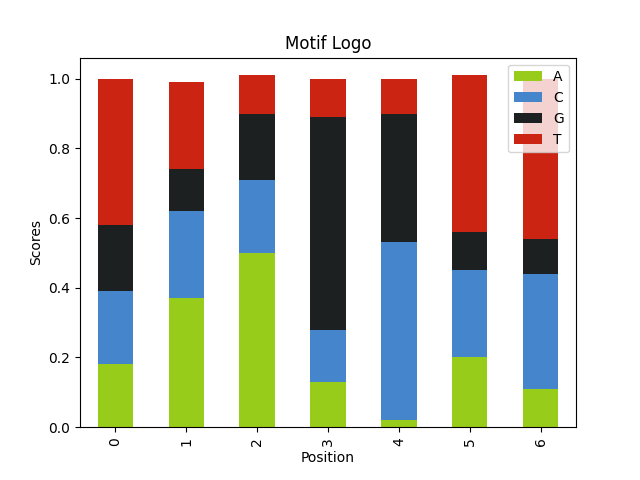### 2.3. Parameters affecting the results

There are few variables that affect the results. Which we can test by tweaking parameters above.

• Length of the motif Longer motifs are easier to find.

Here we have a motif with double the size.

```alphabet = ['A', 'C', 'G', 'T']
seq_weights = [.25, .25, .25, .25]
seq_length = 60
num_seqs = 120

motif_logo = 'AKCYTSG' * 2
motif_weights = [
,
[0.8, 0.2],
,
[0.8, 0.2],
,
[0.8, 0.2],
,
,
[0.8, 0.2],
,
[0.8, 0.2],
,
[0.8, 0.2],
,
]

motif_seqs = []
for i in range(num_seqs):
seq = "".join(
random.choices(population=alphabet, weights=seq_weights, k=seq_length)
)
motif_seq = generate_motif(motif_logo, motif_weights)
motif_seqs.append(insert_motif(seq, motif_seq))

query_seqs = [seq for seq in motif_seqs]
wmm = find_motif(query_seqs, len(motif_logo))
plot_logo(wmm, "motif_longer_logo.png")
```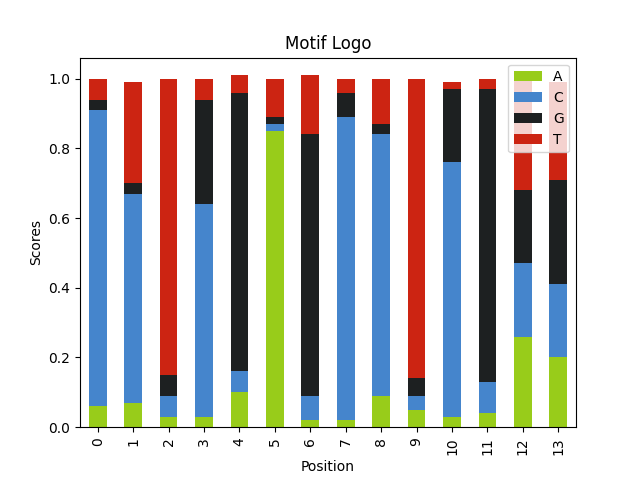• Entropy/information of the motif Motifs with a more conserved sequences are easier to find.

Here we have a motif with lower entropy. Because we change the motif weights to 1, our motif is `AGCCTCG`.

```alphabet = ['A', 'C', 'G', 'T']
seq_weights = [.25, .25, .25, .25]
seq_length = 60
num_seqs = 120

motif_logo = 'AKCYTSG'
motif_weights = [
,
[1, 0],
,
[1, 0],
,
[1, 0],
,
]

motif_seqs = []
for i in range(num_seqs):
seq = "".join(
random.choices(population=alphabet, weights=seq_weights, k=seq_length)
)
motif_seq = generate_motif(motif_logo, motif_weights)
motif_seqs.append(insert_motif(seq, motif_seq))

query_seqs = [seq for seq in motif_seqs]
wmm = find_motif(query_seqs, len(motif_logo))
plot_logo(wmm, "motif_lowentropy_logo.png")
```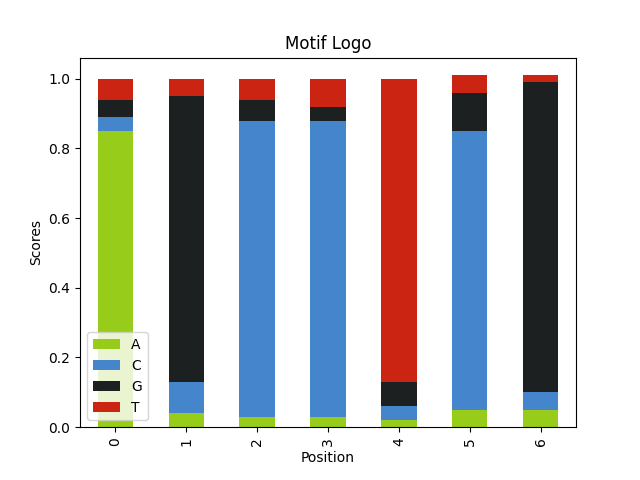• Contrast with the background it is easier to find an A-T rich motif in a G-C rich sequence.

Here we have GC rich sequences with AT rich motifs. Even though our motif is shorther it is much easier to find.

```alphabet = ['A', 'C', 'G', 'T']
seq_weights = [.1, .4, .4, .1]
seq_length = 60
num_seqs = 120

motif_logo = 'AWWT'
motif_weights = [
,
[0.8, 0.2],
[0.8, 0.2],
,
]

motif_seqs = []
for i in range(num_seqs):
seq = "".join(
random.choices(population=alphabet, weights=seq_weights, k=seq_length)
)
motif_seq = generate_motif(motif_logo, motif_weights)
motif_seqs.append(insert_motif(seq, motif_seq))

query_seqs = [seq for seq in motif_seqs]
wmm = find_motif(query_seqs, len(motif_logo))
plot_logo(wmm, "motif_bgcontrast_logo.png")
```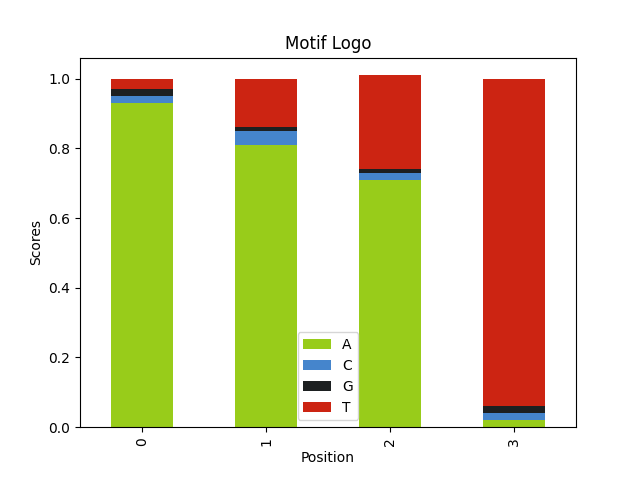## 3. Trying it out with real data

Lets try to use our new program with real data. Download human genome and annotation file.

```wget https://s3-us-west-2.amazonaws.com/human-pangenomics/T2T/CHM13/assemblies/analysis_set/chm13v2.0.fa.gz
wget http://ftp.ebi.ac.uk/pub/databases/ensembl/hprc/y1_freeze/GCA_009914755.4/GCA_009914755.4_genes.gff3.gz
```

We are going to extract 60 bases around end of the 1000 random protein coding exons. First need to get the exon end position for these genes from the annotation file. Easiest way to achieve is with a shell script like below:

1. zcat uncompresses the file
2. grep -v skips the header lines
3. grep ENSP gets annotations with only the proteins
4. after that we get the exon entries with positive orientation and print them with the chromosome and the end position.
5. shuf to randomly sample the whole genome.
6. awk to get chromosome (with chr prefix) start and end positions.
7. lastly head to get first 1000 entries and write them to a file.
```zcat GCA_009914755.4_genes.gff3.gz | grep -v '^#' | grep "ENSP" | awk -F"\t" '{if (\$3 = "exon" && \$7 == "+") {print \$1, \$5}}' | sort -s -k1,1 -k2,2n -u | shuf | awk '{printf  "chr%s:%s-%s\n", \$1, \$2-10, \$2+49}' | head -n 1000 > sample_positions.txt
```

To extract the actual sequences from the genome we are going to use samtools. After indexing the genome `faidx` can retrieve sequences with given coordinates. We can have samtools read the samplepositions.txt file we created and write the outputs to `sample.fa`

```samtools faidx chm13v2.0.fa.gz
samtools faidx chm13v2.0.fa.gz -r sample_positions.txt -o sample.fa
```

We need to read the fasta file.

```with open("sample.fa") as f:
query_seqs = ["".join(entry.splitlines()[1:]).upper() for entry in f.read().split('>')[1:]]
# query_seqs = [line for line f.read().splitlines() if not line.startswith(">")]
print(query_seqs[:12])
```
```['ACATCGAGGAGTGCGTGCGGCGGCAACTGAGGGCCACGGGCCGCCCGCCCAGCACGGAGC', 'AGAACCAGAAGGGTAAGATTACATGTGGGCATAAATTGTTAAAAGCATAGTTATAATGAT', 'CTCAAGGCCAGCGAGCCGGGACTCTTCTCGGTGGGTCTGCACACGGGCGAGGTGCGCACG', 'CGGGCTGCGAGGTAAGAGCGCGCGACCCGCAGCGGCAGATGCACGAACCAGAACGGCCGG', 'ATGTCTATAAGGTGAGCGCCCCCCGGCGCCGAGCTGAGCCCGCTCCGTGTGCGCCCGGGT', 'CCAGGATCCAGGTGAGGGCCCGCTGCGTTCGCAAGTGCGCGCTGGAGCGGAGGCGCTGCG', 'CATCTGCTCAGGTGGGCCTTCAAGAACTTGGGCTCACTCTCTTGGGGTGGAGTTTGCTCC', 'CAAACAAGATGGTAAGTGTCAAAGGAAAATGGCTCCAGATAGAATAAAGGAGGCAAAGAA', 'TTGAAATCAGGGTAAGATGCGAAGCTGGTCGGCCAGGCCAAGGTCTACGACCAGAGTCTG', 'GACATTTAACGGTGAGGTGTATGTTTTATAATTATGTTACCTTCTTAGAAGTGTATTTTT', 'ACTTAGCCAAGGTGAGCTTCTTACCCCGTCCAGGCAGGACCCTAATCCTGGAGCTAGGCA', 'CCCCTCTCTGTGTGAGTATGGGGACCGCTCTCTGTCAGATGCTCTACCAGCAGCAGGGGG']
```

We can just pass the sequences to our function. For the motif size lets pick 8.

```wmm, max_indices = find_motif(random.choices(query_seqs, k=100), 8)
plot_logo(wmm, "5prime_splice_site.png")
```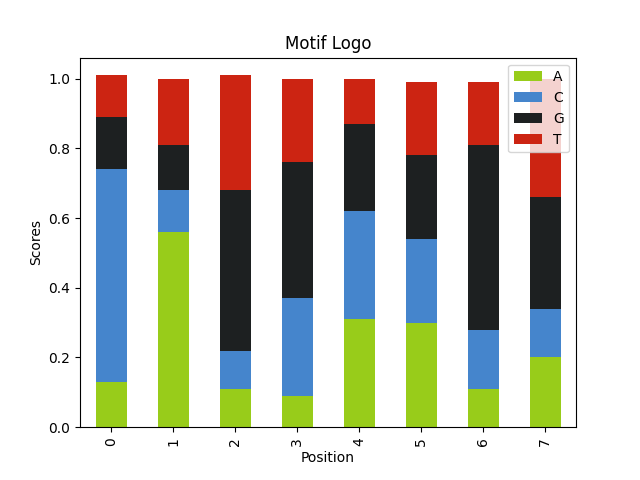Here what we are looking for is the 5´ splice sites with the motif `G-G-[cut]-G-U-R-A-G-U`.